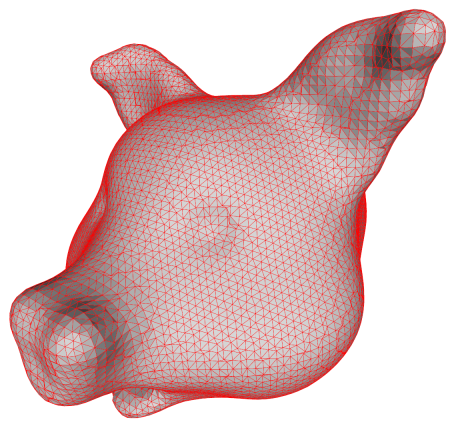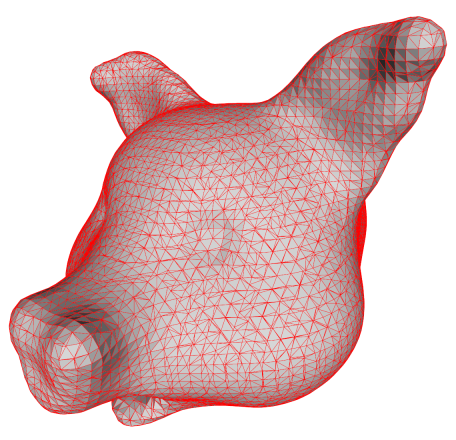Isosurface Polygonization

This page contains brief descriptions of various techniques for polygonizing isosurfaces, i.e. surfaces which are a level-set of some function f(x,y,z). To be precise, the surface is the set of points {(x,y,z) | t=f(x,y,z)} for some value t. t is usually called the isovalue.

There are many methods for isosurface polygonization, and I do not intend to give a survey. The goal of this page is to illustrate some of the better known methods and demonstrate how they are connected.

I am focusing on methods appropriate for volume data and do not consider even obvious optimizations. My main interest here is to show what kinds of meshes that are produced.

The Original

The original model is a distance field which has been volume sculpted using my sculpting tool. A plain isosurface for t=0 is shown below.A "cuberille" Mesh

One of the first ideas for visualizing volumes was to draw each voxel as a small cube. If we do that we get something like the result shown below.It is quite easy to get the mesh above, if we simply find every voxel with a value greater than 0 (remember t=0) and draw a cube, but it is boring. Instead we can select only those cube faces which separate cubes that are inside (t<0) from cubes that are outside (t>=0).

The faces are subsequently stitched together to form a quad mesh. However, sometimes four faces meet at an edge and in this case we must decide which pair to stitch together. This is done using the asymptotic decider by Nielson and Hamann. It was invented to decide ambiguous configurations in marching cubes but it is precisely the same problem.

Dual Contouring

Who says that the vertices have to lie at the cube corners. If we place a cube around each vertex as in the method above, we obtain a 3D grid (whose nodes are the cube vertices) which is precisely the dual of the grid whose nodes are the voxels (i.e. cube centers). Thus, the cuberille method is in a sense the same as dual contouring - originally called Surface Nets by Sarah Frisken. However, when doing dual contouring, we project the vertices onto the surface like you see below:This pushing is often done by moving a vertex along the gradient direction till it hits the isosurface. Here, a slightly improved algorithm is used which tries to find the point on the isosurface which is closest to the original cube corner.

We usually need triangles, so we split the faces into triangles. Doing so naively produces an ugly triangle mesh! Good results are obtained if we triangulate each quad by splitting it along the shortest diagonal as shown below.Marching Cubes

Another thing we can do is to compute the dual of the cuberille mesh. In other words, we place a vertex in the centre of each face and connect with the vertices on the neighbouring faces. Since all faces are quads, this produces a mesh where every vertex is connected to four other vertices. These new vertices don't have to be placed on the face centre. Note that the centre of the face is precisely where the lines of the voxel grid cross the faces of the cubes produced by the cuberille method. Thus, we can use the same method normally used in implementations of marching cubes: We find the point on the line segment between the two voxels sharing the cube face where the isosurface intersects the line segment.

The result which is shown below is, in fact, the precise same result as would have been produced by marching cubes except that the faces have not been polygonized.When we triangulate the faces, we get the same ugly result that we are used to from MC. The uglyness is due to the fact that the vertex placement is more restricted than in dual contouring.Fortunately, we can improve the mesh by collapsing very short edges as shown below. The mesh is still ugly, but the degeneracies (edges of length zero or almost) have been removed.Remarks

It does seem that dual contouring produces nicer meshes than MC but, of course, this depends on the precise method used for placing vertices. Also a naive triangulation of the quad mesh produced by dual contouring will produce strange results.

Marching cubes produces ugly triangles which is well known, but these degeneracies can be fixed easily by edge collapses.

In conclusion, if triangle quality is a concern, the most important thing is probably to use a method which produces a connected mesh and not just a set of triangles (a triangle soup) since in this case, we can use edge collapses and edge flips to nicify the result.

Andreas Bćrentzen
jab 'at' imm.dtu.dk
November 2005